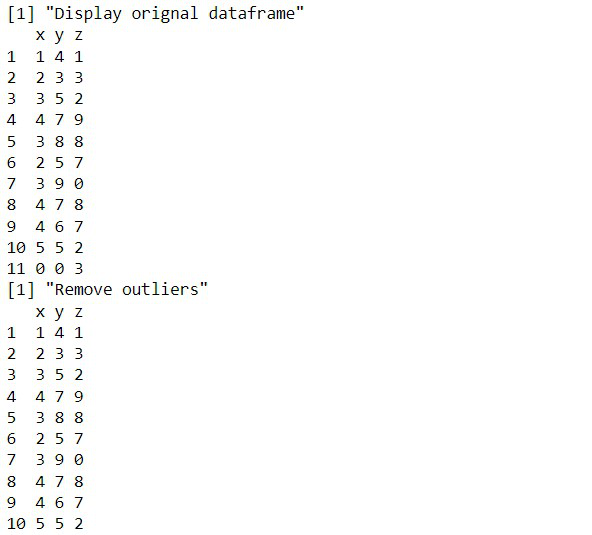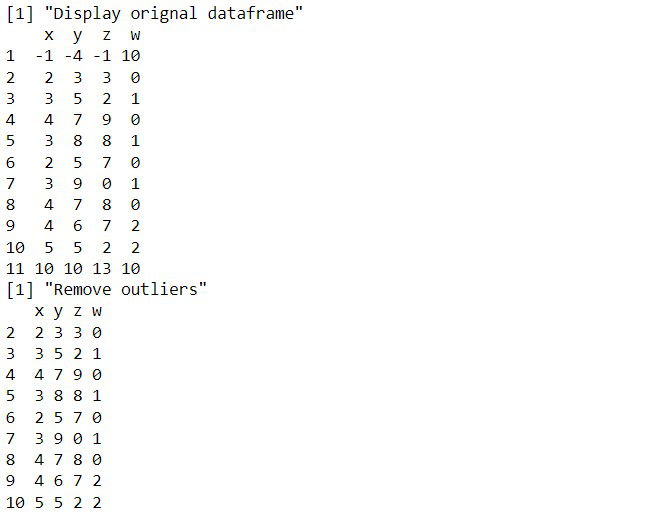Open in App
Not now

# How to Remove Outliers from Multiple Columns in R DataFrame?

• Difficulty Level : Hard
• Last Updated : 03 Feb, 2022

In this article, we will discuss how to remove outliers from Multiple Columns in the R Programming Language.

To remove outliers from a data frame, we use the Interquartile range (IQR) method. This method uses the first and third quantile values to determine whether an observation is an outlier to not. If an observation is 1.5 times the interquartile range greater than the third quartile or 1.5 times the interquartile range less than the first quartile it is considered an outlier.

## Remove Outliers from Multiple Columns in R

To find an outlier in the R Language we use the following function, where we first calculate the first and third quantile of the observation by using the quantile() function. Then we calculate their difference as interquartile range. Then, if an observation is 1.5 times the interquartile range greater than the third quartile or 1.5 times the interquartile range less than the first quartile it returns true.

Syntax:

detect_outlier <- function(x) {

Quantile1 <- quantile(x, probs=.25)

Quantile3 <- quantile(x, probs=.75)

IQR = Quantile3-Quantile1

x > Q3 + (iqr*1.5) | x < Q1 – (iqr*1.5) }

Then once the outlier is identified we remove the outlier by testing them with the above function.

### Example 1:

Here, is an example, where we remove outliers from three columns of the data frame.

## R

 `# create sample data frame` `sample_data < - ``data.frame``(x=``c``(1, 2, 3, 4, 3, 2, 3, 4, 4, 5, 0),` `                           ``y=``c``(4, 3, 5, 7, 8, 5, 9, 7, 6, 5, 0),` `                           ``z=``c``(1, 3, 2, 9, 8, 7, 0, 8, 7, 2, 3))` `print``(``"Display original dataframe"``)` `print``(sample_data)`   `# create detect outlier function` `detect_outlier < - ``function``(x) {`   `    ``# calculate first quantile` `    ``Quantile1 < - ``quantile``(x, probs=.25)`   `    ``# calculate third quantile` `    ``Quantile3 < - ``quantile``(x, probs=.75)`   `    ``# calculate inter quartile range` `    ``IQR = Quantile3-Quantile1`   `    ``# return true or false` `    ``x > Quantile3 + (IQR*1.5) | x < Quantile1 - (IQR*1.5)` `}`   `# create remove outlier function` `remove_outlier < - ``function``(dataframe,` `                            ``columns=``names``(dataframe)) {`   `    ``# for loop to traverse in columns vector` `    ``for ``(col ``in` `columns) {`   `        ``# remove observation if it satisfies outlier function` `        ``dataframe < - dataframe[!``detect_outlier``(dataframe[[col]]), ]` `    ``}`   `    ``# return dataframe` `    ``print``(``"Remove outliers"``)` `    ``print``(dataframe)` `}`   `remove_outlier``(sample_data, ``c``(``'x'``, ``'y'``, ``'z'``))`

Output:### Example 2:

Here, is an example, where we remove outliers from four columns of the data frame.

## R

 `# create sample data frame` `sample_data < - ``data.frame``(x=``c``(-1, 2, 3, 4, 3, 2, 3, 4, 4, 5, 10),` `                           ``y=``c``(-4, 3, 5, 7, 8, 5, 9, 7, 6, 5, 10),` `                           ``z=``c``(-1, 3, 2, 9, 8, 7, 0, 8, 7, 2, 13),` `                           ``w=``c``(10, 0, 1, 0, 1, 0, 1, 0, 2, 2, 10))` `print``(``"Display original dataframe"``)` `print``(sample_data)`     `# create detect outlier function` `detect_outlier < - ``function``(x) {` `  `  `    ``# calculate first quantile` `    ``Quantile1 < - ``quantile``(x, probs=.25)` `  `  `    ``# calculate third quantile` `    ``Quantile3 < - ``quantile``(x, probs=.75)` `  `  `    ``# calculate inter quartile range` `    ``IQR = Quantile3-Quantile1` `  `  `    ``# return true or false` `    ``x > Quantile3 + (IQR*1.5) | x < Quantile1 - (IQR*1.5)` `}`   `# create remove outlier function` `remove_outlier < - ``function``(dataframe,` `                            ``columns=``names``(dataframe)) {` `  `  `    ``# for loop to traverse in columns vector` `    ``for ``(col ``in` `columns) {` `      `  `        ``# remove observation if it satisfies outlier function` `        ``dataframe < - dataframe[!``detect_outlier``(dataframe[[col]]), ]` `    ``}` `  `  `    ``# return dataframe` `    ``print``(``"Remove outliers"``)` `    ``print``(dataframe)` `}`   `remove_outlier``(sample_data, ``c``(``'x'``, ``'y'``, ``'z'``, ``'w'``))`

Output:My Personal Notes arrow_drop_up
Related Articles